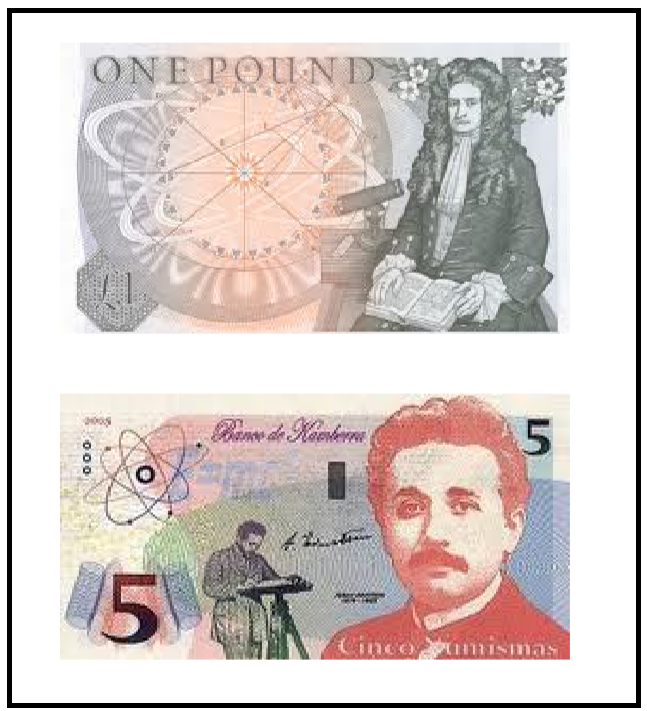### ACM 20050 Classical Mechanics and Special RelativityI will be teaching this module in 2022-2023.

Description: This course starts by developing the principles of Newtonian Mechanics for particles, systems of particles and rigid bodies. It focuses on applications of Newton's laws with an emphasis on problem solving. It then introduces the fundamental concept of Einstein's theory of Special Relativity and the relativistic extension of Newtonian concepts such as energy relativity including Einstein's famous formula E=mc^2.

Topics and techniques discussed include but are not limited to the following list:

• Newton's laws of motion: Revision of Newton's laws of motion and the concept of kinetic and potential energy. Conservative force fields and conservation of energy. Equilibrium of a particle and stability of equilibrium.
• Central forces and planetary and satellite motion: Motion in a plane is discussed with a focus on central forces. Newton's law of gravitation. Determination of the orbit from the central force. Kepler's laws of planetary motion and the motion of satellites.
• Moving coordinate systems: Non-inertial coordinate systems. Rotating coordinate systems. Velocity and acceleration in a moving frame. Coriolis and centripetal acceleration.
• Systems of Particles: Centre of mass. Momentum of a system of particles. Motion of the centre of mass. Angular momentum of a system of particles. Total external moment acting on a system and it relation to the angular momentum.
• Einstein's Special Theory of Relativity: Einstein's basic postulates of special relativity. The Lorentz transformation and the concept of simultaneity. Length contraction, time dilation, and elative velocity explained through the concepts of space-time diagrams and 4-vectors. The concepts of inertial mass, energy and momentum in special relativity including E=mc^2.

Learning Outcomes: On completion of this module students should be able to:

1. Analyse the stability of equilibrium positions of a particle
2. Solve orbit problems in mathematical terms
3. Explain the concepts of planetary and satellite orbits including Kepler's laws
4. Derive expressions for the velocity and acceleration in a rotating frame
5. Explain the concepts of angular velocity, angular momentum for a system of particle
6. Understand relation to the angular momentum and total external moment acting on a system
7. Describe Einstein's postulates of special relativity and derive the Lorentz transformation
8. Explain the geometrical interpretation of the Lorentz transformations in terms of space-time diagrams and the associated concept of 4-vector.
9. Derive the results of special relativity on simultaneity, length contraction, time dilation and relative velocity. 10. Explain the equivalence of mass and energy.

### Module Documents

The pandemic has made us all rely more on proprietary technologies, owned by faceless unaccountable bureucratic corporations. This module is no exception and for that reason, the module will be delivered via the UCD Virtual Learning Environment for the year 2022-2023. However, the most up-to-date version of the lecture notes (from 2020) is made available here as a resource.